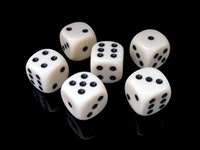## Dice Probability

### The What

Where to begin? This isn't so much a project as a decades-long hobby. From my childhood onward, I've enjoyed gaming. From board games we all know and love, to role-playing games, to dice games…I've enjoyed a broad interest in a variety of games. And many of them utilize dice as a means of introducing chance.

### The Why

I like to know the how of things; why they work the way they do. Knowing how something is designed, knowing why it functions the way it does, is just what my brain seeks out.

I've dabbled in game design, whether designing whole-cloth, or fixing aspects of game designs of others for my personal use. As random chance is a core element of many games, and dice being a broadly used method of randomization in gaming, I took to understanding the dice better as I got deeper into game design.

Sure, cards can and are used. Coins as well. But for some reason I had an affinity for dice, and other than collecting them and using them in games, I wanted to better understand their function as a randomizing element in game design.

### The Outcome

The most evident outcome of all this research comes in the form of code I have written to automate the calculations of the combinations, distributions of frequency, and probabilities of complex dice mechanics for games.

In 2005, I released Games::Dice::Probability, a PERL module released to CPAN providing a simplified way of parsing complex dice expressions and calculating them.

In 2021, I've released diceprob, a Golang module for performing the same parsing and calculations as G::D::P did.

### The Crunch; Let's Get Nerdy!

#### Permutations

A roll of some dice is, mathematically, a permutation with repetition. That is, each dice has s sides, and each die can roll to present a single number from [1,s], and multiple of them can display the same number in a single roll.

Since repetition is allowed, you have s sides per die, and it can combine with each of the other dice with s sides. Therefore, the number of permutations p for a number of dice n with each die having the same number of sides s is represented as:

$$p=s_1 \cdot s_2 \cdot \dotsc \cdot s_n = s^n$$

#### Bounds

Now that we know the total number of permutations p, we can define our total of the die faces t, bounded between a minimum:

$$t_{min}=n$$

and a maximum:

$$t_{max}=ns$$

giving a bounds of t:

$$t\in[t_{min},t_{max}]$$

#### Probability

So we can calculate the probability of any dice sum total of t with:

$$P(t,n,s)=\frac{1}{p} \sum_{k=0}^{\left\lfloor{\frac{t-n}{s}}\right\rfloor} (-1)^k \binom{n}{k} \binom{t-sk-1}{n-1}$$

#### Distribution

So to build the distribution, you simply would solve P(t,n,s) for every value of t. This can be computationally expensive as n and s grow.

Luckily, dice outcomes represent a normal distribution, which has symmetry around µ, which is the mean, median, and mode for the distribution. So we only need to solve t from minimum to µ.

$$\mu = \left\lfloor \frac{n (s + 1)}{2} \right\rfloor = \left\lfloor \frac{ns + n}{2} \right\rfloor = \left\lfloor \frac{t_{max} + t_{min}}{2} \right\rfloor$$

Once we've calculated P(t,n,s) from n to µ, we can remove half of the calculation overhead and define the symmetric P(t,n,s) values for t from µ to maximum with the following identity:

$$P(t_1,n,s) \equiv P(t_2,n,s)$$

where:

$$t_2 = t_{max} + t_{min} - t_1$$

Alternatively, for a total t or higher on n dice of s sides:

$$P(\geq t,n,s)=\frac{1}{p} \sum_{k=0}^{\left\lfloor{\frac{t-n}{s}}\right\rfloor} (-1)^k \binom{n}{k} \binom{t-sk-1}{t-sk-n}$$

or:

$$P(\geq t,n,s)=\frac{1}{p} \sum_{k=0}^{n} (-1)^k \binom{n}{k} \binom{t-sk}{t-sk-n}$$

Or, for a total t or lower on n dice of s sides:

$$P(\leq t,n,s)=\frac{1}{p} \sum_{k=0}^{\left\lfloor{\frac{t-n-1}{s}}\right\rfloor} (-1)^k \binom{n}{k} \binom{t-sk-1}{n}$$

### Random Notes

Some other dice methods and their equations. For possible use in coding projects.

1. Where nDs won't have repetition, solve for p with (not really a thing? better represented as some other dice construct?):

$$p=\binom{ \left(s+n-1\right) }{n}$$

2. “Middle” dice is a concept where you roll three dice and take the middle value. Commonly saw it in use in game design to more closely approximate the distribution of 3dX in place of 1dX mechanics. For example, the 1d20 flat distribution of D&D and variants can be mitigated by doing mid20. Solve for P(t,s) from 1 to µ (and complete the distribution with P(t1,s)==P(t2,s)), with:

$$P(t,s)=\frac{1}{p}(1 + 3(s-1) + 6(t-1)(s-t))$$

3. Second highest, where t is the second highest face value on n dice of s sides:

$$P(t,n,s)=\frac{1}{p}(t^n+n(t^{(n-1)})(s-t))$$

4. Face values, where exactly d dice of t face value when rolling n dice of s sides:

$$P(d,t,n,s)=\frac{1}{p}(s-1)^{(n-d)}\binom{n}{d}$$

5. Face values, where exactly d dice of t face value or higher when rolling n dice of s sides:

$$P(d,t,n,s)=\frac{1}{p}(t-1)^{(n-d)}(s-t+1)^d\binom{n}{d}$$

6. Face values, where exactly d dice of t face value or lower when rolling n dice of s sides:

$$P(d,t,n,s)=\frac{1}{p}(s-t)^{(n-1)}t^d\binom{n}{d}$$